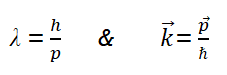Goseeko blog# What are Matter waves?

## Matter waves

According to the De-Broglie concept, with each moving particle a wave is associated. These associated waves are called matter waves.

That is, the wave–particle duality present in light must also occur in matter. So, starting from the momentum of a photon p = h ν /c = h/λ, we can generalize this relation to any material particle with non zero rest mass. Each material particle of momentum  behaves as a group of waves whose wavelength λ and wave vector  are governed by the speed and mass of the particle.Wave has wavelength  λ here h is Planck’s constant and p is the momentum of the moving particle.

## Properties

• As already discussed, the wave associated with matter in motion is Matter wave. Wavelength is λ=h/p.
• These are localized waves, not a progressive.
• This is a group of waves also known as a wave packet. These wave packet are form due to the superposition of two or more progressive waves. Thus these are not single waves at all.
• As these represent the direction of propagation of matter, So we also call it with the name of pilot wave.
• The speed of matter wave depends on the mass of the particle. We know electromagnetic wave moves with the speed of light but this case is not valid for matter wave. Matter waves do not have constant speed.
• Vg x Vp= c2 shows that Vp, phase velocity of matter wave is greater than velocity of light. This shows that these are not physical waves.
• As mass is inversely proportional to the wavelength. So for lighter particles, the De-Broglie wavelength is greater.
• As velocity is inversely proportional to the wavelength. So for faster particle moves, the smaller is its De-Broglie wavelength.
• The De-Broglie wavelength of a particle is independent of the charge or nature of the particle.
• Only charged particles can produce the electromagnetic wave. The matter wave is not electromagnetic in nature.

Interested in learning about similar topics? Here are a few hand-picked blogs for you!